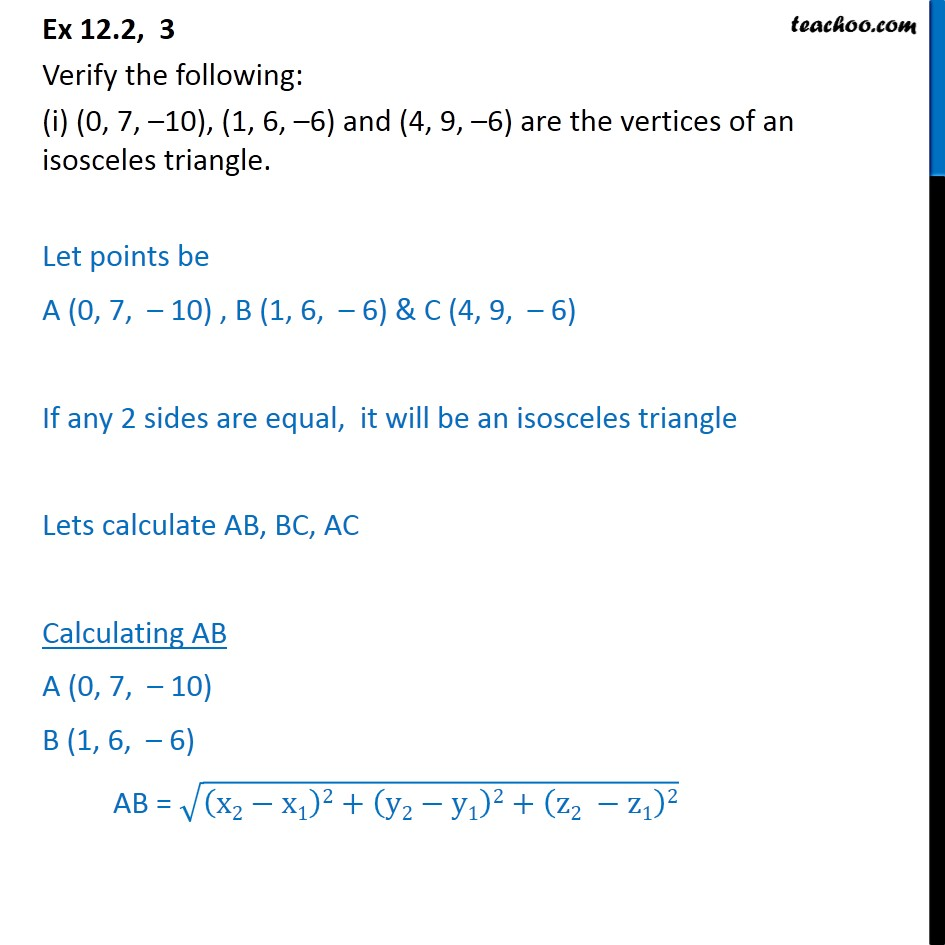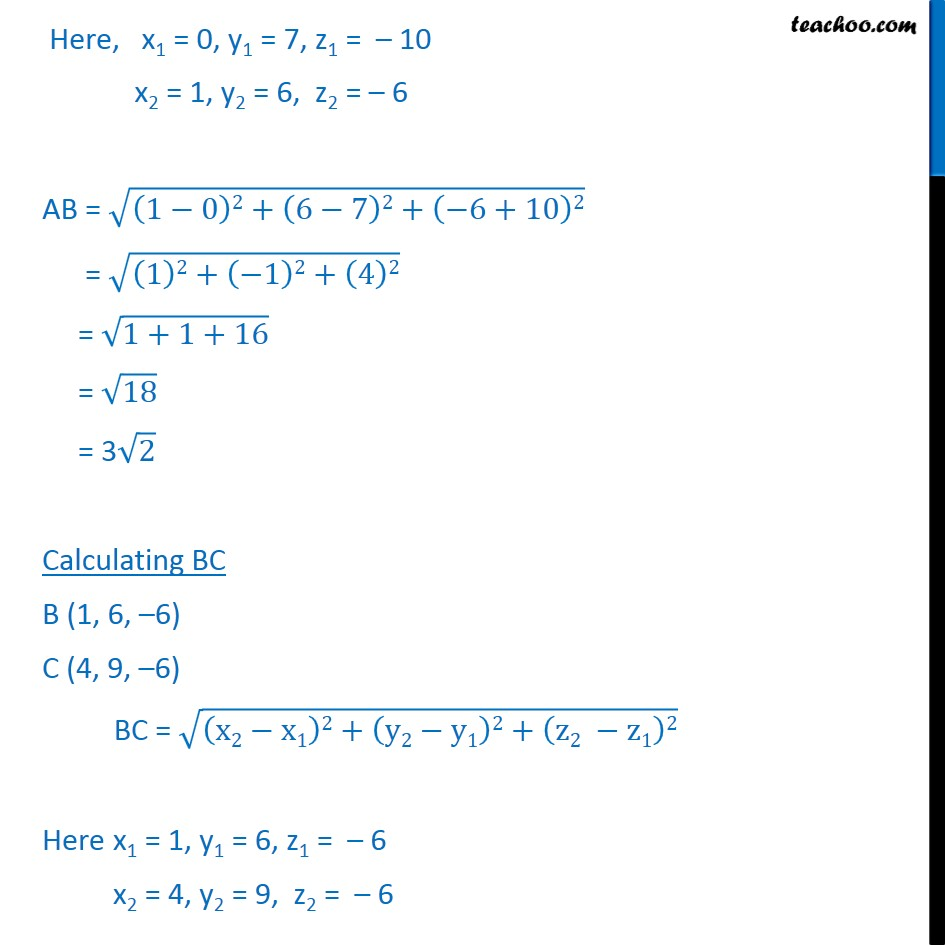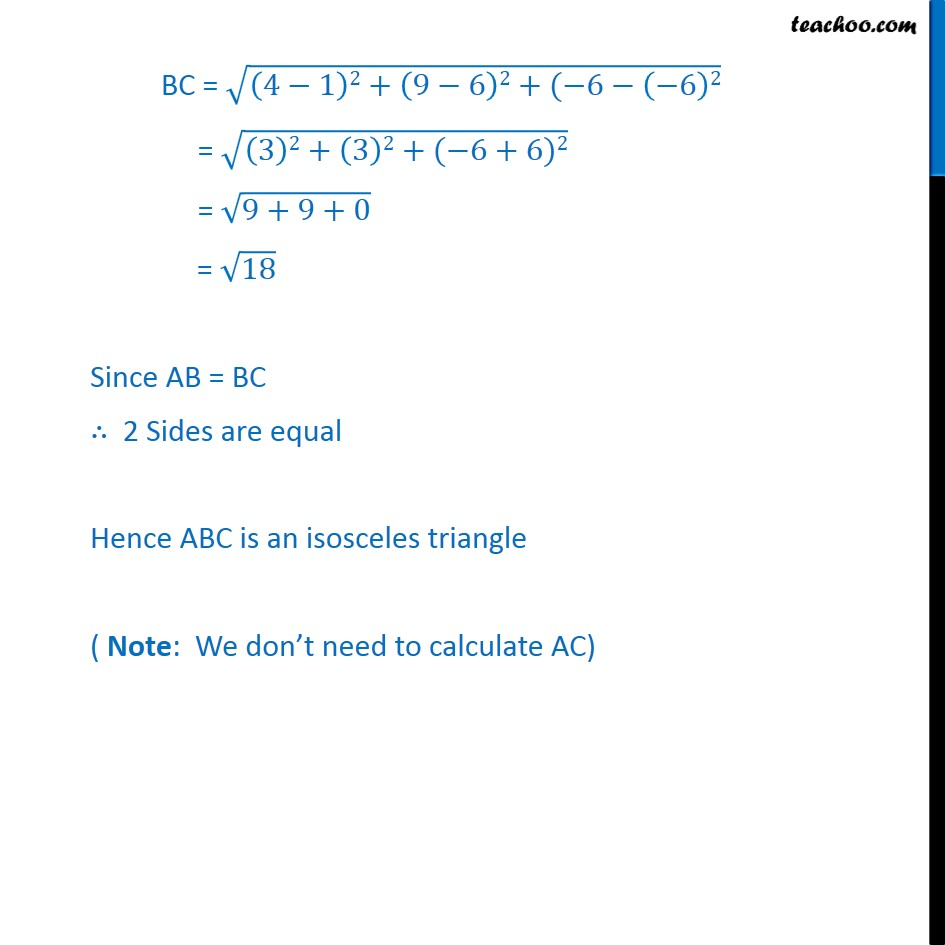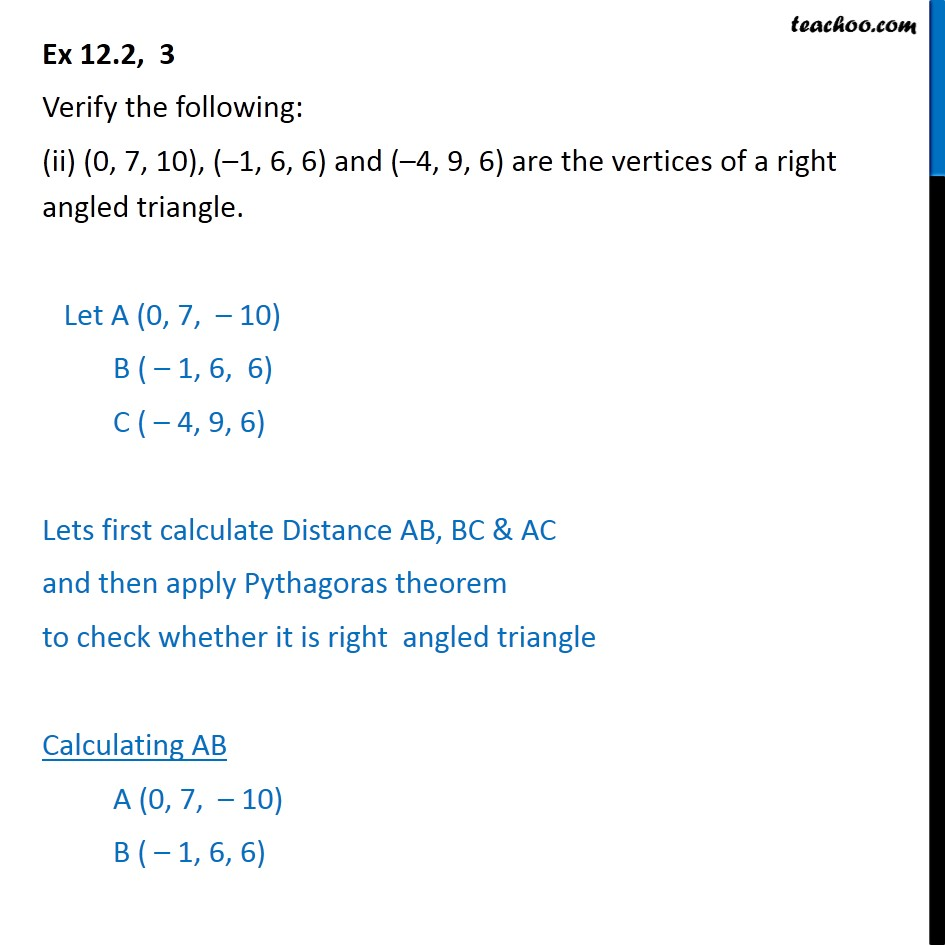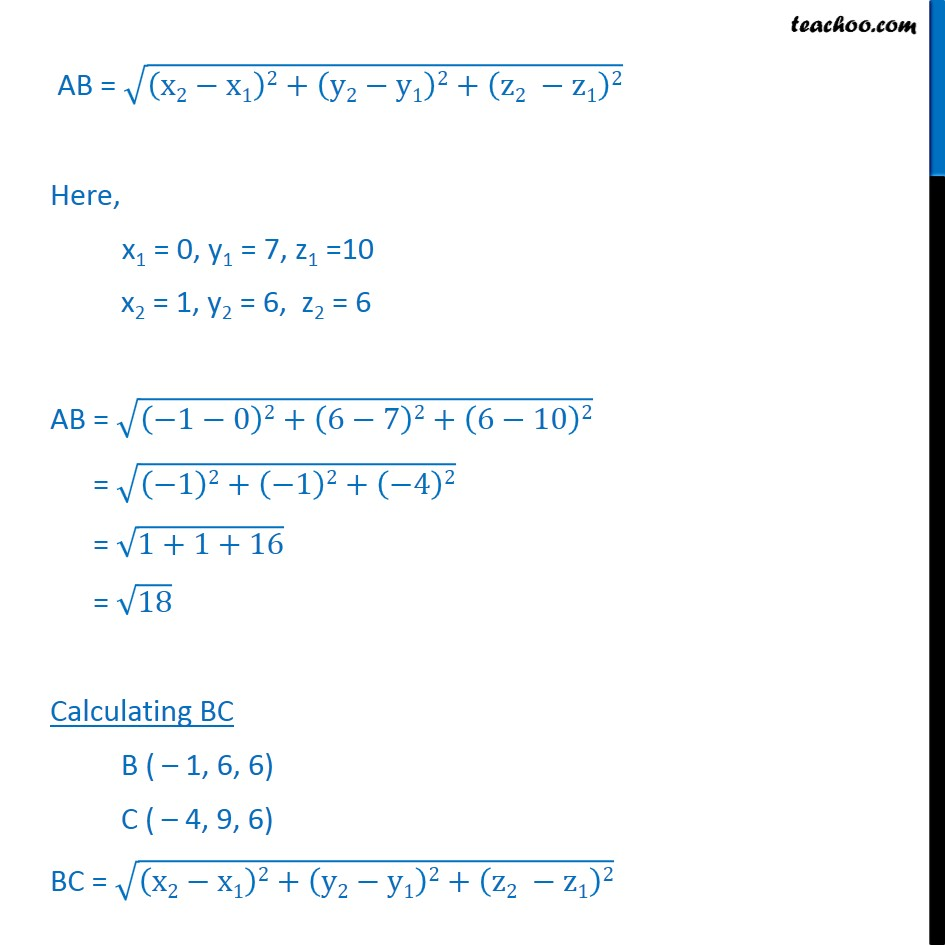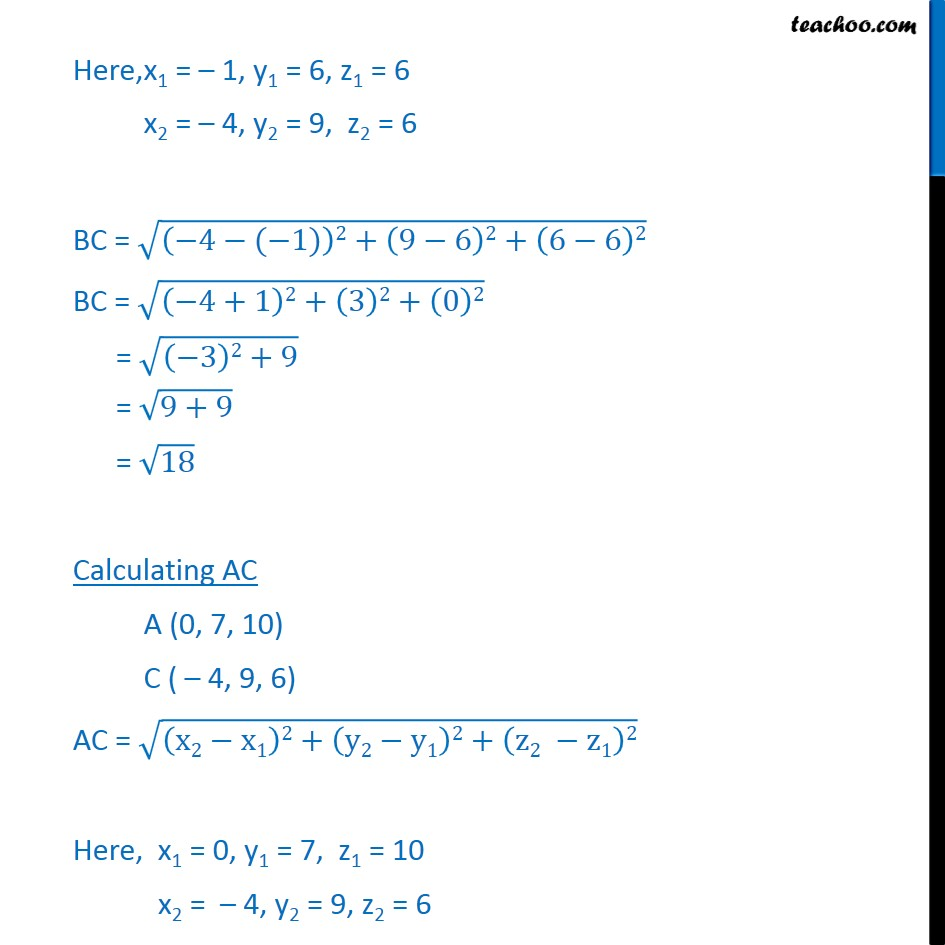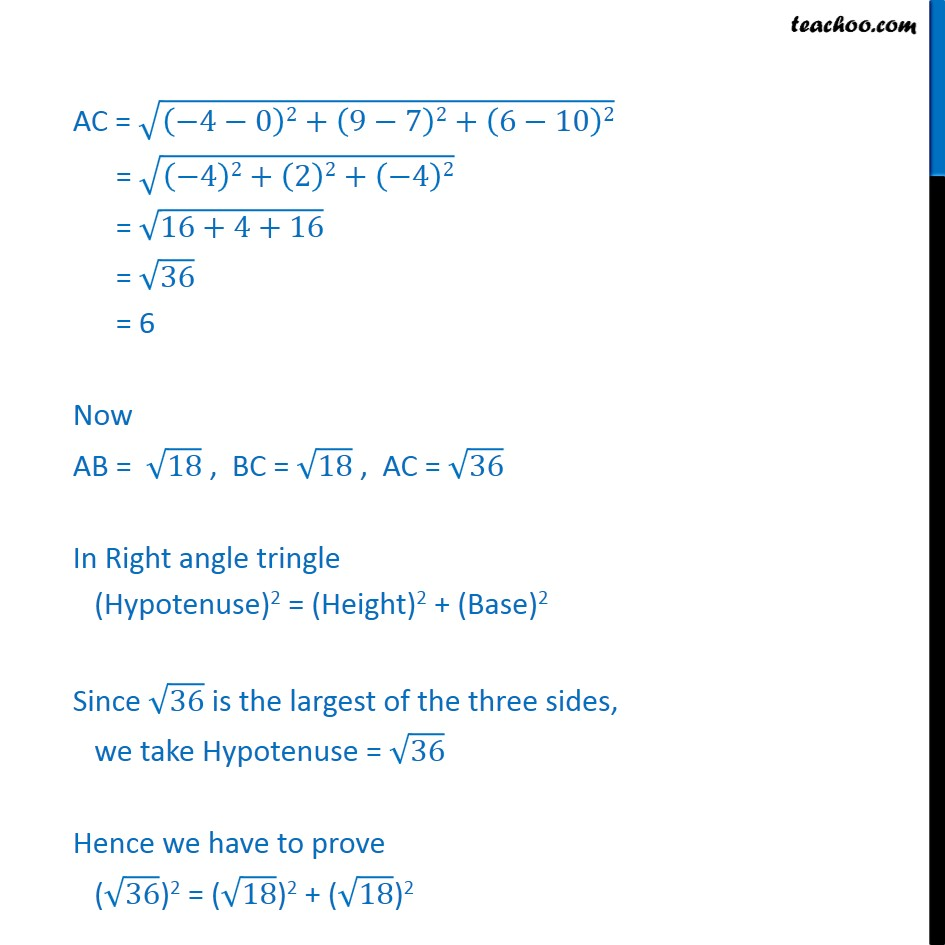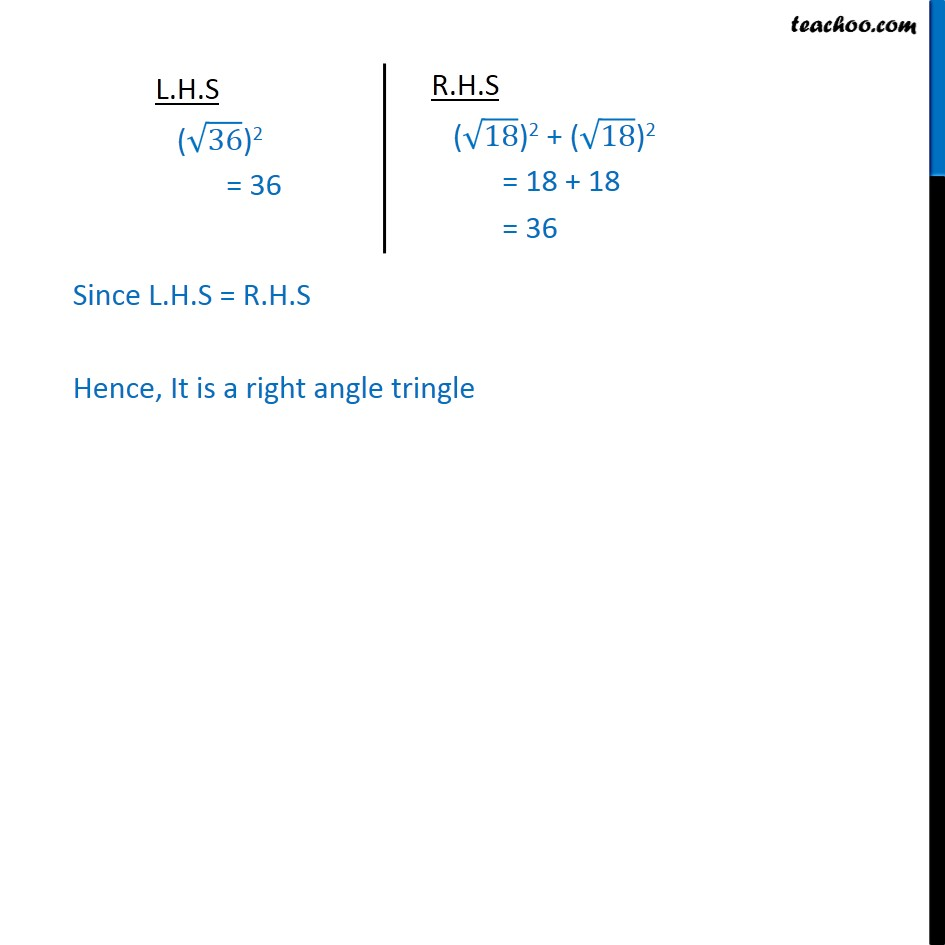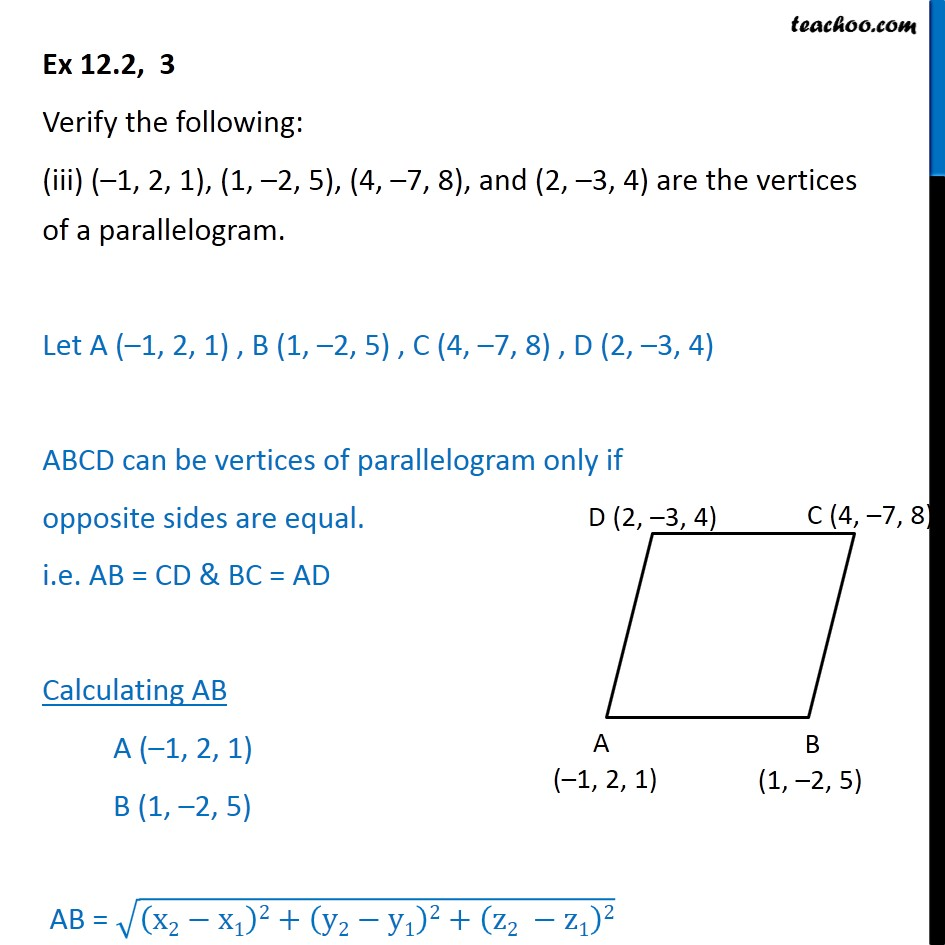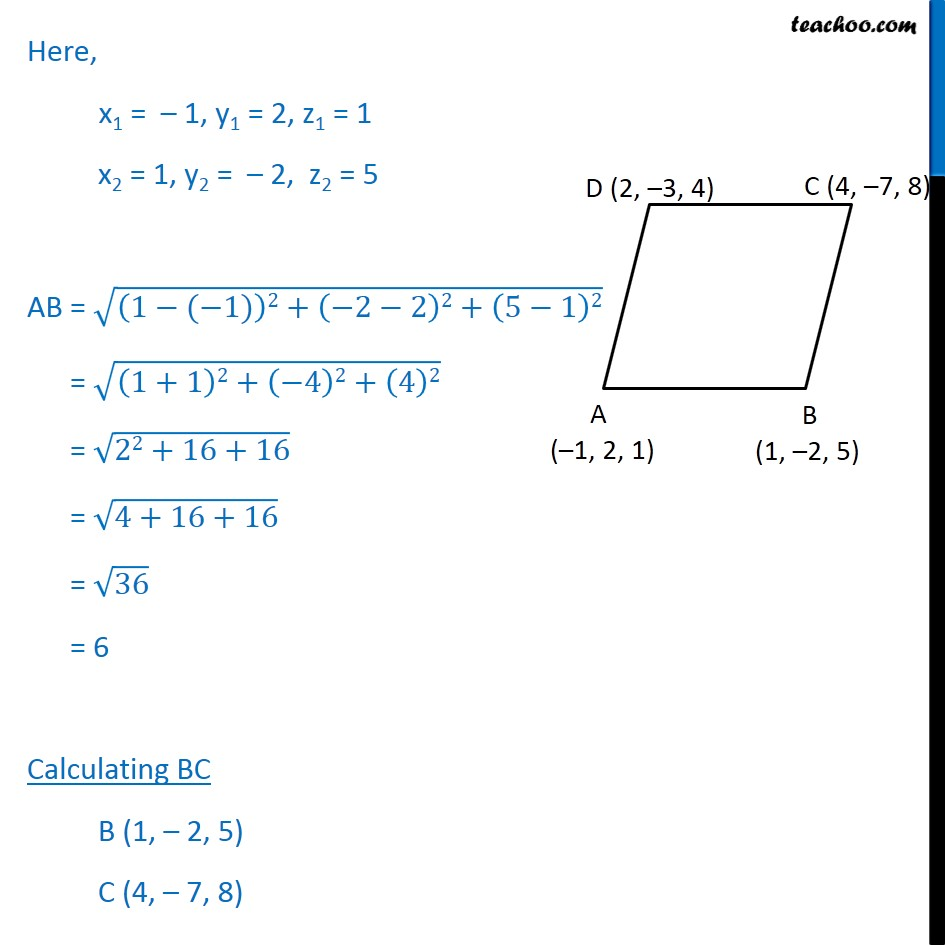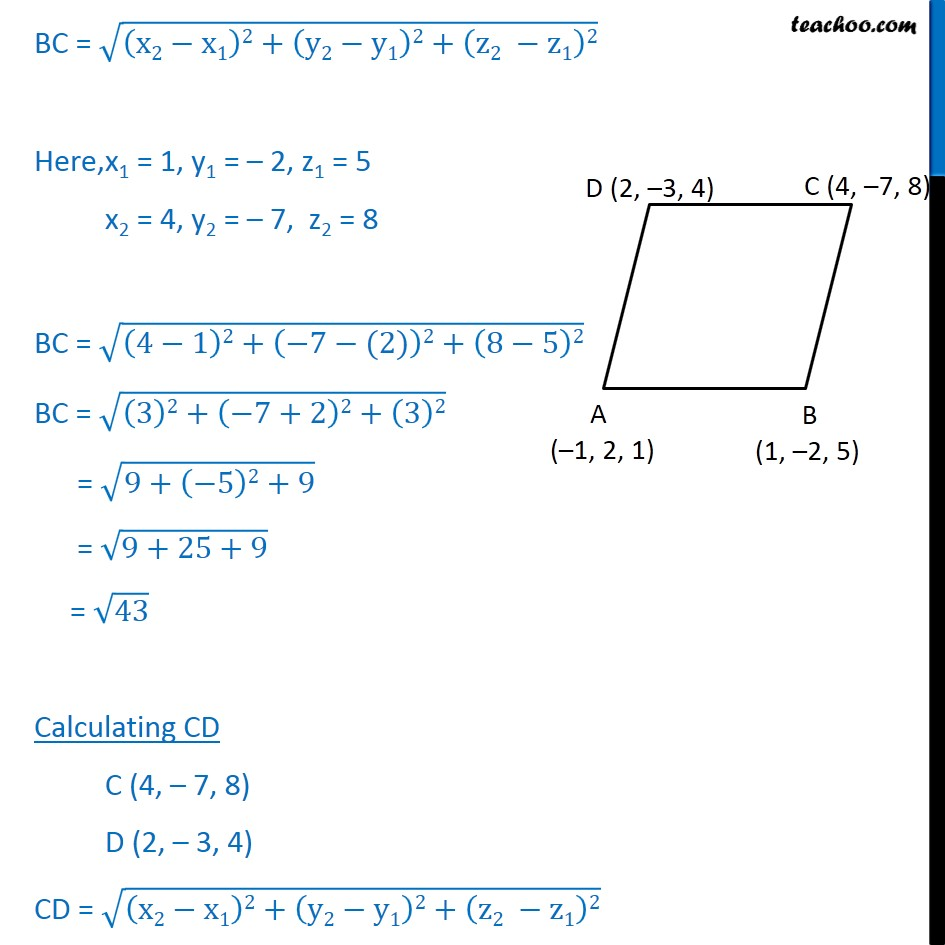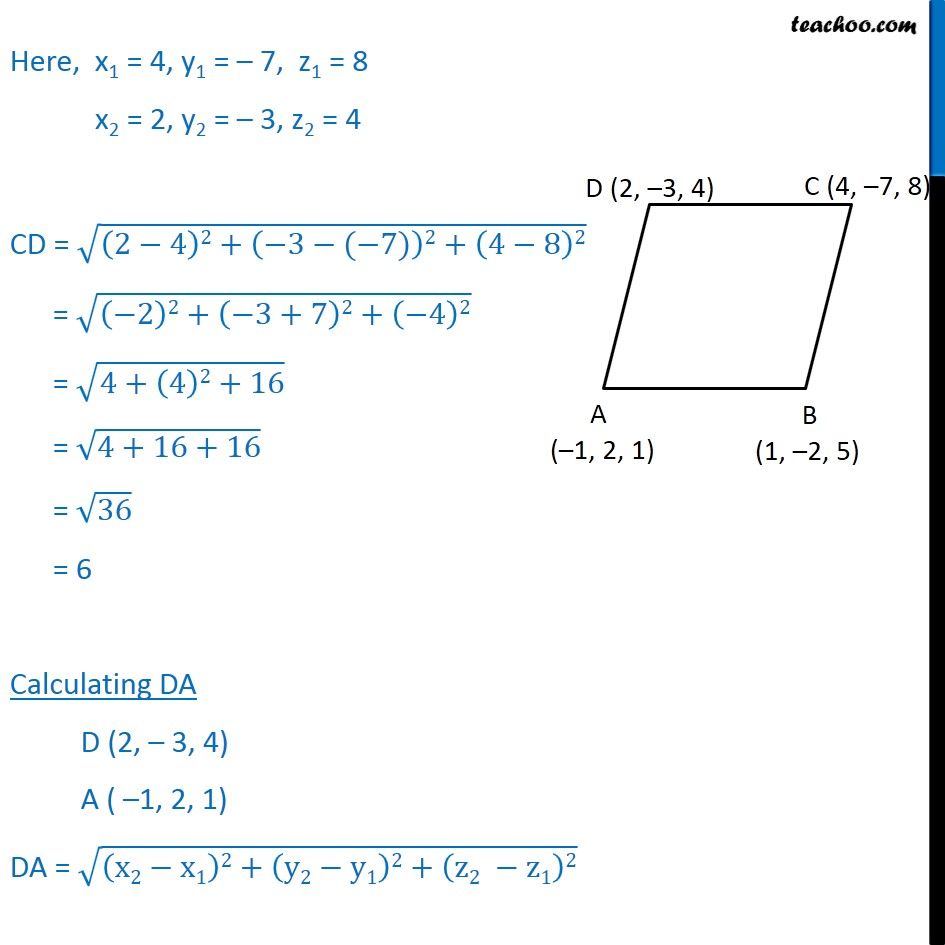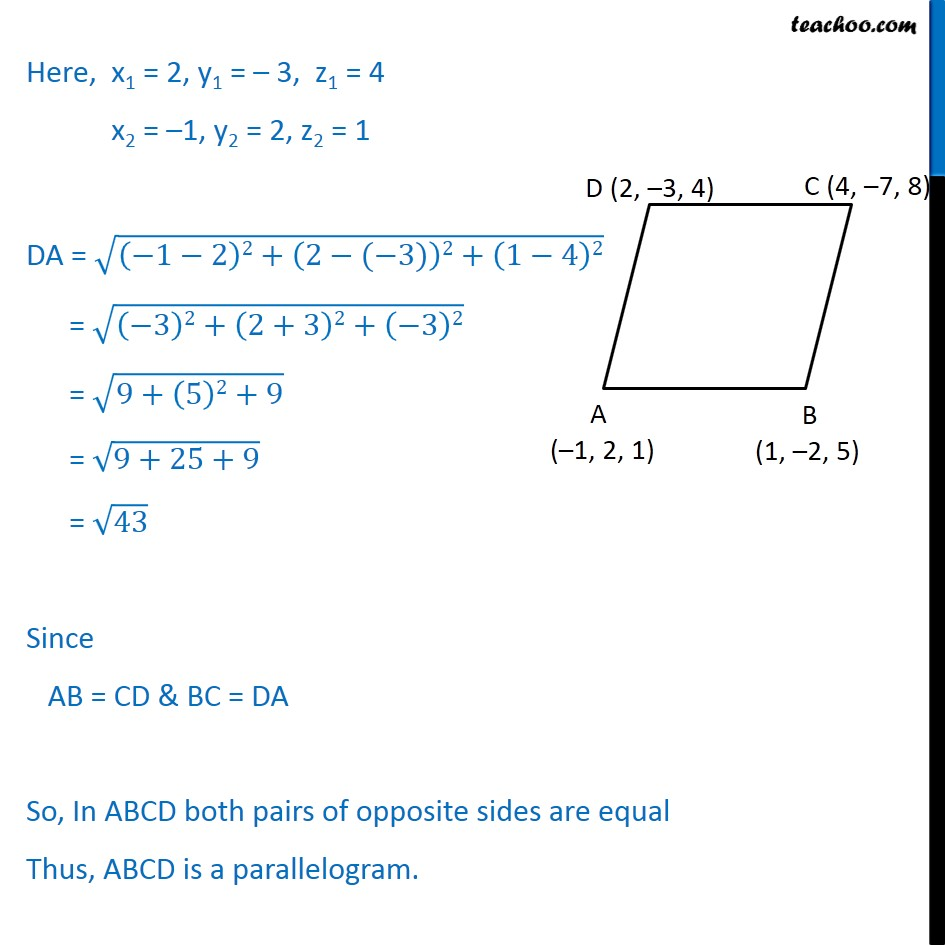1. Chapter 12 Class 11 Introduction to Three Dimensional Geometry
2. Serial order wise
3. Ex 12.2

Transcript

Ex 12.2, 3 Verify the following: (i) (0, 7, 10), (1, 6, 6) and (4, 9, 6) are the vertices of an isosceles triangle. Let points be A (0, 7, 10) , B (1, 6, 6) & C (4, 9, 6) If any 2 sides are equal, it will be an isosceles triangle Lets calculate AB, BC, AC Calculating AB A (0, 7, 10) B (1, 6, 6) AB = x2 x1 2+ y2 y1 2+ z2 z1 2 Here, x1 = 0, y1 = 7, z1 = 10 x2 = 1, y2 = 6, z2 = 6 AB = 1 0 2+ 6 7 2+ 6+10 2 = 1 2+ 1 2+ 4 2 = 1+1+16 = 18 = 3 2 Calculating BC B (1, 6, 6) C (4, 9, 6) BC = x2 x1 2+ y2 y1 2+ z2 z1 2 Here x1 = 1, y1 = 6, z1 = 6 x2 = 4, y2 = 9, z2 = 6 BC = 4 1 2+ 9 6 2+( 6 6 2 = 3 2+ 3 2+( 6+6)2 = 9+9+0 = 18 Since AB = BC 2 Sides are equal Hence ABC is an isosceles triangle ( Note: We don t need to calculate AC) Ex 12.2, 3 Verify the following: (ii) (0, 7, 10), ( 1, 6, 6) and ( 4, 9, 6) are the vertices of a right angled triangle. Let A (0, 7, 10) B ( 1, 6, 6) C ( 4, 9, 6) Lets first calculate Distance AB, BC & AC and then apply Pythagoras theorem to check whether it is right angled triangle Calculating AB A (0, 7, 10) B ( 1, 6, 6) AB = x2 x1 2+ y2 y1 2+ z2 z1 2 Here, x1 = 0, y1 = 7, z1 =10 x2 = 1, y2 = 6, z2 = 6 AB = 1 0 2+ 6 7 2+ 6 10 2 = 1 2+ 1 2+ 4 2 = 1+1+16 = 18 Calculating BC B ( 1, 6, 6) C ( 4, 9, 6) BC = x2 x1 2+ y2 y1 2+ z2 z1 2 Here, x1 = 1, y1 = 6, z1 = 6 x2 = 4, y2 = 9, z2 = 6 BC = 4 ( 1) 2+ 9 6 2+ 6 6 2 BC = 4+1 2+ 3 2+ 0 2 = 3 2+9 = 9+9 = 18 Calculating AC A (0, 7, 10) C ( 4, 9, 6) AC = x2 x1 2+ y2 y1 2+ z2 z1 2 Here, x1 = 0, y1 = 7, z1 = 10 x2 = 4, y2 = 9, z2 = 6 AC = 4 0 2+ 9 7 2+ 6 10 2 = 4 2+ 2 2+ 4 2 = 16+4+16 = 36 = 6 Now AB = 18 , BC = 18 , AC = 36 In Right angle tringle (Hypotenuse)2 = (Height)2 + (Base)2 Since 36 is the largest of the three sides, we take Hypotenuse = 36 Hence we have to prove ( 36 )2 = ( 18 )2 + ( 18 )2 Since L.H.S = R.H.S Hence, It is a right angle tringle Ex 12.2, 3 Verify the following: (iii) ( 1, 2, 1), (1, 2, 5), (4, 7, 8), and (2, 3, 4) are the vertices of a parallelogram. Let A ( 1, 2, 1) , B (1, 2, 5) , C (4, 7, 8) , D (2, 3, 4) ABCD can be vertices of parallelogram only if opposite sides are equal. i.e. AB = CD & BC = AD Calculating AB A ( 1, 2, 1) B (1, 2, 5) AB = x2 x1 2+ y2 y1 2+ z2 z1 2 Here, x1 = 1, y1 = 2, z1 = 1 x2 = 1, y2 = 2, z2 = 5 AB = 1 ( 1) 2+ 2 2 2+ 5 1 2 = 1+1 2+ 4 2+ 4 2 = 22+16+16 = 4+16+16 = 36 = 6 Calculating BC B (1, 2, 5) C (4, 7, 8) BC = x2 x1 2+ y2 y1 2+ z2 z1 2 Here, x1 = 1, y1 = 2, z1 = 5 x2 = 4, y2 = 7, z2 = 8 BC = 4 1 2+ 7 (2) 2+ 8 5 2 BC = 3 2+ 7+2 2+ 3 2 = 9+ 5 2+9 = 9+25+9 = 43 Calculating CD C (4, 7, 8) D (2, 3, 4) CD = x2 x1 2+ y2 y1 2+ z2 z1 2 Here, x1 = 4, y1 = 7, z1 = 8 x2 = 2, y2 = 3, z2 = 4 CD = 2 4 2+ 3 ( 7) 2+ 4 8 2 = 2 2+ 3+7 2+ 4 2 = 4+ 4 2+16 = 4+16+16 = 36 = 6 Calculating DA D (2, 3, 4) A ( 1, 2, 1) DA = x2 x1 2+ y2 y1 2+ z2 z1 2 Here, x1 = 2, y1 = 3, z1 = 4 x2 = 1, y2 = 2, z2 = 1 DA = 1 2 2+ 2 ( 3) 2+ 1 4 2 = 3 2+ 2+3 2+ 3 2 = 9+ 5 2+9 = 9+25+9 = 43 Since AB = CD & BC = DA So, In ABCD both pairs of opposite sides are equal Thus, ABCD is a parallelogram.

Ex 12.2